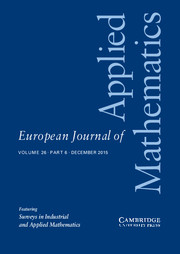HomeEuropean Journal of Applied Mathematics

# The unified method for the heat equation: I. non-separable boundary conditions and non-local constraints in one dimension

Published online by Cambridge University Press:  18 July 2013

Corresponding

## Abstract

We use the heat equation as an illustrative example to show that the unified method introduced by one of the authors can be employed for constructing analytical solutions for linear evolution partial differential equations in one spatial dimension involving non-separable boundary conditions as well as non-local constraints. Furthermore, we show that for the particular case in which the boundary conditions become separable, the unified method provides an easier way for constructing the relevant classical spectral representations avoiding the classical spectral analysis approach. We note that the unified method always yields integral expressions which, in contrast to the series or integral expressions obtained by the standard transform methods, are uniformly convergent at the boundary. Thus, even for the cases that the standard transform methods can be implemented, the unified method provides alternative solution expressions which have advantages for both numerical and asymptotic considerations. The former advantage is illustrated by providing the numerical evaluation of typical boundary value problems.

## Keywords

Type
Papers
Information
European Journal of Applied Mathematics , December 2013 , pp. 857 - 886

## Access options

Get access to the full version of this content by using one of the access options below.

## References

Cohen, D. S. (1966) An integral transform associated with boundary conditions containing an eigenvalue parameter. J. SIAM Appl. Math. 14 (5), 11641175.CrossRefGoogle Scholar
Deconinck, B., Trogdon, T. & Vasan, V. (2013) The method of Fokas for solving linear partial differential equations. SIAM Rev. (accepted).Google Scholar
Dujardin, G. M. (2009) Asymptotics of linear initial-boundary value problems with periodic boundary data on the half-line and finite intervals. Proc. R. Soc. Lond. Ser. A 465, 33413360.CrossRefGoogle Scholar
Flyer, N. & Fokas, A. S. (2008) A hybrid analytical-numerical method for solving evolution partial differential equations: I. The half-line. Proc. R. Soc. Lond. Ser. A 464, 18231849.CrossRefGoogle Scholar
Fokas, A. S. (1997) A unified transform method for solving linear and certain nonlinear PDEs. Proc. R. Soc. Lond. Ser. A 453, 14111443.CrossRefGoogle Scholar
Fokas, A. S. (2000) On the integrability of linear and nonlinear PDEs. J. Math. Phys. 41, 41884237.CrossRefGoogle Scholar
Fokas, A. S. (2002) A new transform method for evolution of partial differential equations. IMA J. Appl. Math. 67, 559590.CrossRefGoogle Scholar
Fokas, A. S. & Pelloni, B. (2000) Integral transforms, spectral representation and the d-bar problem. Proc. R. Soc. Lond. Ser. A Math. Phys. Eng. Sci. 456, 805833.CrossRefGoogle Scholar
Fokas, A. S. & Spence, E. A. (2012) Synthesis as opposed to separation of variables. SIAM Rev. 54, 291324.CrossRefGoogle Scholar
Fokas, A. S. & Sung, L. Y. (1999) Initial-Boundary Value Problems for Linear Dispersive Evolution Equations on the Half-Line. (Unpublished manuscript, Department of Mathematics. Imperial College. London).Google Scholar
Fokas, A. S. & Treharne, P. A. (2007) Initial-boundary value problems for linear PDEs with variable coefficients. Math. Proc. Camb. Phil. Soc. 143, 221242.Google Scholar
Mugnolo, D. & Nicaise, S. (2013) Well-posedness and spectral properties of heat and wave equations with non-local conditions. J. Differ. Equ. (accepted) arXiv:1112.0415.Google Scholar
Pelloni, B. (2005) The spectral representation of two-point boundary-value problems for third-order linear evolution partial differential equations. Proc. R. Soc. Lond. Ser. A Math. Phys. Eng. Sci. 461, 2965.CrossRefGoogle Scholar
Pelloni, B. & Smith, D. A. (2013) Spectral theory of some non-self-adjoint linear differential operators. Proc. R. Soc. Lond. Ser. A Math. Phys. Eng. Sci. 469, 2154.CrossRefGoogle Scholar
Smith, D. A. (2012) Well-posed two-point initial-boundary value problems with arbitrary boundary conditions. Math. Proc. Camb. Phil. Soc. 152, 473496.CrossRefGoogle Scholar

### Full text views

Full text views reflects PDF downloads, PDFs sent to Google Drive, Dropbox and Kindle and HTML full text views.

Total number of HTML views: 0
Total number of PDF views: 60 *
View data table for this chart

* Views captured on Cambridge Core between September 2016 - 27th January 2021. This data will be updated every 24 hours.

Hostname: page-component-898fc554b-gwqw7 Total loading time: 0.581 Render date: 2021-01-27T11:07:04.134Z Query parameters: { "hasAccess": "0", "openAccess": "0", "isLogged": "0", "lang": "en" } Feature Flags: { "shouldUseShareProductTool": true, "shouldUseHypothesis": true, "isUnsiloEnabled": true, "metricsAbstractViews": false, "figures": false, "newCiteModal": false }

# Send article to Kindle

Note you can select to send to either the @free.kindle.com or @kindle.com variations. ‘@free.kindle.com’ emails are free but can only be sent to your device when it is connected to wi-fi. ‘@kindle.com’ emails can be delivered even when you are not connected to wi-fi, but note that service fees apply.

Find out more about the Kindle Personal Document Service.

The unified method for the heat equation: I. non-separable boundary conditions and non-local constraints in one dimension
Available formats
×

# Send article to Dropbox

To send this article to your Dropbox account, please select one or more formats and confirm that you agree to abide by our usage policies. If this is the first time you use this feature, you will be asked to authorise Cambridge Core to connect with your <service> account. Find out more about sending content to Dropbox.

The unified method for the heat equation: I. non-separable boundary conditions and non-local constraints in one dimension
Available formats
×

# Send article to Google Drive

To send this article to your Google Drive account, please select one or more formats and confirm that you agree to abide by our usage policies. If this is the first time you use this feature, you will be asked to authorise Cambridge Core to connect with your <service> account. Find out more about sending content to Google Drive.

The unified method for the heat equation: I. non-separable boundary conditions and non-local constraints in one dimension
Available formats
×
×